NEET  >  Addition & Subtraction of Vectors (Triangular & Parallelogram Laws) - Graphical Method

# Addition & Subtraction of Vectors (Triangular & Parallelogram Laws) - Graphical Method - Physics Class 11 - NEET

4. LAWS OF ADDITION AND SUBTRACTION OF VECTORS

4.1 Triangle rule of addition: Steps for addicting two vector representing same physical quantity by triangle law.

(i) Keep vectors s.t. tail of one vector coincides with head of others.

(ii) Join tail of first to head of the other by a line with arrow at head of the second.

(iii) This new vector is the sum of two vectors. (also called resultants)

(i)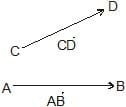(ii)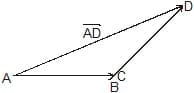(iii)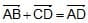Take example here.

Q. A boy moves 4 m south and then 5 m in direction 37° E of N. Find resultant displacement.

4.2 Polygon Law of addition :

This law is used for adding more than two vectors. This is extension of triangle law of addition. We keep on arranging vectors s.t. tail of next vector lies on head of former.

When we connect the tail of first vector to head off last we get resultant of all the vectors.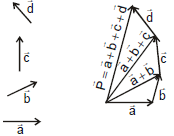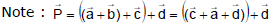[Associative Law]

4.3 Parallelogram law of addition :

Steps :

(i) Keep two vectors such that their tails coincide.

(ii) Draw parallel vectors to both of them considering both of them as sides of a parallelogram.

(iii) Then the diagonal drawn from the point where tails coincide represents the sum of two vectors, with its tail at point of coincidence of the two vectors.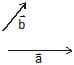(i)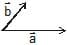(ii)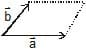(iii)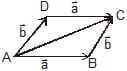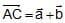Note: and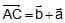thus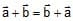[Commutative Law]

Note: Angle between 2 vectors is the angle between their positive directions.

Suppose angle between these two vectors is θ, and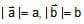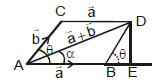= (AB + BE)2 + (DE)2

= (a +b cosθ)2 + (b sinθ)

= a2 + b2 cos2θ + 2ab cosθ + b2 sin2θ

= a2 + b2 + 2ab cosθ

Thus, AD =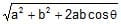or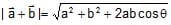angle α with vector a is

tan α =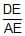=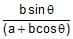Important points :

•  To a vector, only a vector of same type can be added that represents the same physical quantity and the resultant is also a vector of the same type.
•  As R = [A2 + B2 + 2AB cosθ]1/2 so R will be maximum when, cosθ = max = 1,

i.e., θ = 0º, i.e. vectors are like or parallel and Rmax = A + B.

•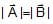and angle between them θ then R =•and angle between them π -θ then R =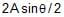•  The resultant will be minimum if, cosθ = min = -1, i.e., θ = 180º, i.e. vectors are antiparallel and Rmin = A -B.
•  If the vectors A and B are orthogonal, i.e., θ = 90º,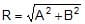•  As previously mentioned that the resultant of two vectors can have any value from (A -B) to (A + B)      depending on the angle between them and the magnitude of resultant decreases as q increases 0º to 180º.
•  Minimum number of unequal coplanar vectors whose sum can be zero is three.
•  The resultant of three non-coplanar vectors can never be zero, or minimum number of non coplanar vectors whose sum can be zero is four.

5. SUBTRACTION OF VECTORS :

Negative of a vector say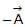is a vector of the same magnitude as vector but pointing in a direction opposite to that of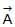.

Thus,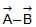can be written as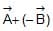oris really the vector addition ofand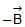.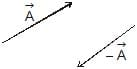Suppose angle between two vectors and is θ. Then angle between andwill be 180° -θ as shown in figure.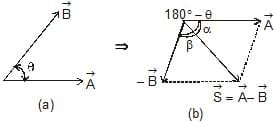Magnitude of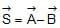will be thus given by

S =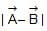=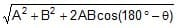or S =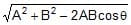...(i)

For direction of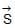we will either calculate angle α or β, where,

tanα =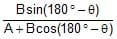=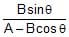...(ii)

or tanβ =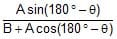=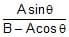...(iii)

Ex.3 Two vectors of 10 units & 5 units make an angle of 120° with each other. Find the magnitude & angle of resultant with vector of 10 unit magnitude.

Sol.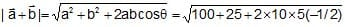=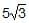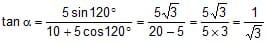⇒ α = 30°

[Here shows what is angle between both vectors = 120° and not 60°]

Note: or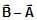can also be found by making triangles as shown in the figure. (a) and (b)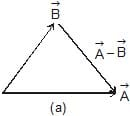Or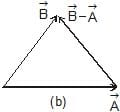Ex.4 Two vectors of equal magnitude 2 are at an angle of 60° to each other find magnitude of their sum & difference.

Sol.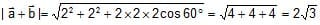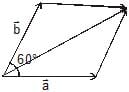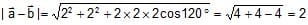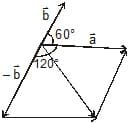Ex.5     Find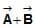and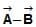in the diagram shown in figure. Given A = 4 units and B = 3 units.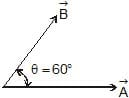R =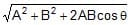=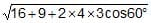=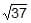units

tanα =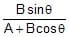=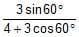= 0.472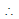a = tan-1(0.472) = 25.3°

Thus, resultant ofand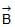isunits at angle 25.3° fromin the direction shown in figure.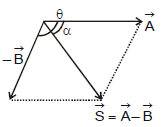Subtraction : S ==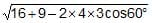=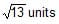and tanθ ==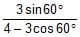= 1.04

∴ α = tan-1 (1.04) = 46.1°

Thus,is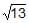units at 46.1° fromin the direction shown in figure.

6. Unit Vector and Zero vector

Unit vector is a vector which has a unit magnitude and points in a particular direction. Any vector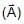can be written as the product of unit vector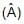in that direction and magnitude of the given vector.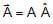or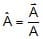A unit vector has no dimensions and unit. Unit vectors along the positive x-, y-and z-axes of a rectangular coordinate system are denoted by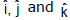and respectively such that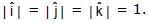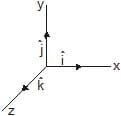A vector of zero magnitudes is called zero or a null vector. Its direction is arbitrary.

Ex.6 A unit vector along East is defined as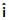. A force of 105 dynes acts west wards. Represent the force in terms ofSol.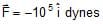The document Addition & Subtraction of Vectors (Triangular & Parallelogram Laws) - Graphical Method | Physics Class 11 - NEET is a part of the NEET Course Physics Class 11.
All you need of NEET at this link: NEET

## Physics Class 11

116 videos|451 docs|188 tests

## FAQs on Addition & Subtraction of Vectors (Triangular & Parallelogram Laws) - Graphical Method - Physics Class 11 - NEET

 1. What is the graphical method of adding and subtracting vectors?Ans. The graphical method of adding and subtracting vectors involves using vector diagrams to visually represent the vectors and determine their resultant vector. The Triangle Law and Parallelogram Law are two common graphical methods used to perform vector addition and subtraction.
 2. How does the Triangle Law work for vector addition?Ans. The Triangle Law states that to add two vectors, you can place them head to tail and draw a vector from the tail of the first vector to the head of the second vector. The resultant vector is the vector drawn from the tail of the first vector to the head of the second vector.
 3. Can you explain the Parallelogram Law for vector addition?Ans. The Parallelogram Law states that to add two vectors, you can construct a parallelogram using the vectors as adjacent sides. The resultant vector is then represented by the diagonal of the parallelogram that starts from the same point as the vectors.
 4. How do you subtract vectors using the graphical method?Ans. To subtract vectors using the graphical method, you can follow the same steps as vector addition. However, when subtracting a vector, you need to reverse its direction before adding it to the other vector using the Triangle Law or Parallelogram Law.
 5. What are some advantages of using the graphical method for vector addition and subtraction?Ans. The graphical method provides a visual representation of vector addition and subtraction, making it easier to understand and analyze vector quantities. It allows for a quick and intuitive way to determine the magnitude, direction, and resultant of vectors. Additionally, the graphical method can be used to verify algebraic calculations and check for errors.

## Physics Class 11

116 videos|451 docs|188 testsExplore Courses for NEET exam### How to Prepare for NEET

Read our guide to prepare for NEET which is created by Toppers & the best Teachers
Signup to see your scores go up within 7 days! Learn & Practice with 1000+ FREE Notes, Videos & Tests.
10M+ students study on EduRev
Track your progress, build streaks, highlight & save important lessons and more!
Related Searches

,

,

,

,

,

,

,

,

,

,

,

,

,

,

,

,

,

,

,

,

,

;